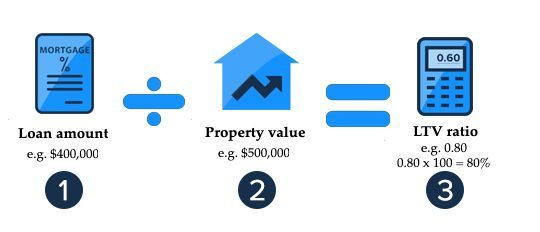# Demystifying Loan-to-Value (LTV) Ratio in Hard Money Loans

Are you a real estate enthusiast or a beginner investor looking for financing options for your next venture? If you’re interested in hard money loans, you will also be interested in the important concept – the loan-to-value (LTV) ratio. This guide will delve into LTV ratios and explain the important applications in affordable credit.

## The loan-to-value (LTV) ratio concept

It is an essential statistical measure of credit risk and is fundamental to credit evaluation. Here’s how the technology works:

• Calculating the LTV ratio
• LTV and Risk
• Impacts on Loan Approval

### 1. Calculating the LTV Ratio:

It is a ratio of critical metrics calculated by dividing the loan amount by the property’s appraised value. The formula is as follows:

For example, The LTV ratio of observing a property valued at \$200,000 and requiring a \$150,000 loan is 75%. That can be calculated as :

LTV Ratio = (Loan Value / Appraised Asset Value) *.

In our example: (150,000 / 200,000) * 100 = 75%.

### 2. LTV and Risk:

The LTV ratio is an essential indicator of the riskiness of high-quality loans. A higher
LTV ratio indicates a higher risk for the lender. If this figure reaches or exceeds 100%, the loan fully covers the property’s value. This can be frustrating for lenders because if the borrower defaults, the lender may not get their deposit.

### 3. Impact on loan approval:

The LTV ratio dramatically determines whether you’ll be approved for a Hard Money loan. Many aggressive lenders prefer lower LTV rates to reduce risk. Lenders may be more willing to approve loans with LTV rates below 70%, but higher LTV rates may require more detailed appraisals and higher interest rates.

### LTV Ratios and Hard Money Loans

Here’s why it’s essential to understand LTV ratios for someone considering a hard money loan:

• Risk Assessment
• Negotiating Power
• Loan Amount Determination

1. Risk assessment:

By considering the LTV ratio, borrowers can assess the level of risk associated with their loan. For a loan with a higher LTV rate, be prepared for potentially higher interest rates and more onerous terms.

2 . Negotiating power:

Knowing your property’s appraised value and LTV ratio can give you an edge when negotiating with aggressive lenders. You will be better prepared to make your case for the right loan.

3. Loan Amount Determination:

The LTV ratio also helps determine the maximum loan amount you can secure. It’s a pivotal factor in understanding how much financing you can obtain based on the value of your property.

## Conclusion

Loan-to-Value (LTV) ratio is a vital cog in the mechanics of hard money loans. Understanding the LTV ratio is not only pivotal in the approval of your loan but also holds significant sway over the terms and interest rates you’ll encounter. As you navigate the realm of hard money loans, a firm grasp of LTV ratios empowers you with the knowledge and confidence needed to make well-informed choices and secure the financing essential for your real estate ventures. So, whether you’re a seasoned investor or a first-time borrower, consider the power of LTV ratios in hard money loans.